## Smith Charts Explained

### Why We Need Smith Charts

In the world of RF (Radio Frequency) electronics, normal “bench-top” circuit components cease to operate the way they were designed to.  This means a normal resistor can become a capacitor, a capacitor can become an inductor, and a normal wire can become a distributed network of inductors and capacitors.  This highly non-ideal behavior occurs because, in reality, no true resistor, capacitor, inductor, or wire exists; rather they are all processed and manufactured to operate within a certain frequency range – at frequencies where the real-world effects are quantitatively insignificant.  However, as one approaches RF frequencies, these real-world effects become much more pronounced in cheap components.   Eventually, the frequency of operation can become so high that the transmission line itself – no longer a simple wire – will exhibit significant signal-loss.  But even with lossless transmission lines, it is important in communications to “match impedances,” i.e. attach an antenna whose impedance matches that of the signal source – this maximizes the transmitting-antenna’s power dissipation (and “reflects” back zero power).  Indeed, being able to calculate and measure the impedances of antennas, transmission lines, etc is very important within RF design, which are almost always complex numbers.  Another reason determining load impedances is important is because of the 1:1 mapping between a value of load impedance and a corresponding value of$\Gamma$, the reflection coefficient (a ratio of how much a signal is reflected versus how much a signal is radiated for a given load).

### How To Read a Smith Chart

One way of simplifying the analytical problems communication engineers typically face is by using a Smith Chart.  Smith Charts provide a graphical representation of the impedance of any load – whether that load be an antenna or simply an open-circuited transmission line, such as a coax cable.  Because these impedances may very well be complex in nature, a Smith Chart is designed such that each point on it represents both the real and imaginary parts of the load’s impedance.   To begin, observe the basic “format” of any Smith Chart:

What is seen here is the generic, normalized Smith Chart.  Each point on the chart represents an impedance, and the numbers marked on the chart represent different coefficients needed to multiply by the original load value  (from which the chart was normalized from – If this is confusing, don’t worry about it – the “original load value” will almost always be known).  The chart consists of yellow circles and red arcs.  The yellow circles represent contours of where the Real part of the impedance magnitude is the same, e.g. for any point along the yellow circle marked “2.0,” the real part of the impedance is: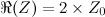where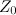is the impedance the chart was normalized from.

Similarly, the red arcs represent contours where the Imaginary part of the impedance magnitude is the same, e.g. for any point along the red arc marked “0.5,” the imaginary part of the impedance is: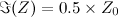This is very handy for displaying complex impedance values.  For instance, notice the following Smith Chart:

What is the impedance for the load represented on the Smith Chart by the blue dot?  This is easy to determine, because:

1. The blue dot is along the yellow circle marked “2.0,” so the real part of the impedance must be: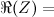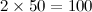2. The blue dot is also along the red arc marked “0.5,” so the imaginary part of the impedance must be: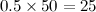So, from this, we have determined that the impedance of the “load” represented by the blue dot is: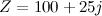By using this method, it is simple to find the impedance represented by any point on the Smith Chart!

### Useful Smith Chart Relations

As mentioned before, a Smith Chart is really just a 1:1 mapping between a value of load impedance and a value of$\Gamma$, the reflection coefficient of a load.  The reflection coefficient is defined as:$\Gamma =\frac{V_{reflected}}{V_{incident}} = \frac{V_{refl}}{V_{inc}}$

The reflection coefficient is a very important metric.  For antennas, a reflection coefficient expresses how much signal voltage is used in exciting the antenna and how much signal voltage is reflected back to the source.  For an ideal antenna,$\Gamma$ would be zero – corresponding to$Z = Z_0 \Omega$, which is the origin of the Smith Chart!  In fact, simple relations exist between$Z$ and$\Gamma$: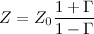and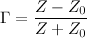Useful Notes

Simple observations allow for a more “intuitive” approach when using Smith Charts.  Note the following:

• The straight red line in the center is an “arc” representing all points where the imaginary part of the impedance is zero
• The furthest-left point on the straight red line represents where the impedance is zero (or, a short circuit), and the furthest-right point on the straight red line represents where the impedance is$\infty$ (or, an open circuit)
• The very top point of the Smith Chart is where the impedance is +$j$
• For this reason, the top half of the Smith Chart represents inductive loads
• The very bottom point of the Smith Chart is where the impedance is –$j$
• Similarly, the bottom half of the Smith Chart represents capacitive loads

____

Safa Khamis

## Circular Buffers

Software circular buffers are data structures used to pass data from one section of code to another, where the code sections usually have no other interaction with each other. One situation that dictates that a “buffer” be used is when it is possible that a burst of data to occur that exceeds the “processing” codes ability to keep up on an item by item basis. Then a separate section of code to buffer (accept from the external source and insert it into a circular buffer) is necessary.

On average, the processing must keep up, or no amount of buffering will prevent an eventual overrun and loss of data. Another situation where you might use a “buffer” is to compartmentalize the functions of your code. For example, if you are using a keypad on a project, you may choose to write one section of code that detects that a key has been pressed, determines what it is, and places the ASCII code for it in a circular buffer.

A completely separate section of code could then process the “stream” of key-presses for the purpose at hand, say a combination lock code. Partitioning the code in this fashion not only makes each section easier to write (each is smaller and you get to focus on a simpler task), but also makes each section more reusable. The combination decoding could easily be used virtually unchanged with the code sequence coming from the SCI instead. The proper view of data in a circular buffer is a “stream” of sequential data items, often characters.

The section of code that sends data to another section inserts each new datum item into the buffer. In general, that code inserts data as fast as it becomes available. The section of code that receives the data removes each item from the buffer.  The receiver almost always has some processing task to perform on the received data items, and often processes at a slower instantaneous rate than the insertion code. It is often the case that several circular buffers will exist within a program. It is also often the case that the sending and/or receiving code sections are in interrupt handler routines. A possible point of confusion is that any receiving ISR routine (say an SCI receive ISR) will probably play the role of sender via the circular buffer. Virtually the only SCI receiver ISR I have ever written was to have it read the incoming characters and place them in a circular buffer, and nothing more.

### How Do Circular Buffers Work?

A circular buffer is really a linear sequential buffer that is used from beginning to end, over and over. Unlike a STACK which operates as a first-in-last-out (FILO) buffer and naturally keeps reusing memory as items are popped on and later pulled off of the stack, with a circular buffer one must work at reusing memory. This is accomplished by having code to wrap around to the beginning of the buffer whenever the next location gets past the end of the buffer. Picture the buffer as a consecutive set of memory locations that has its last location adjacent to its first location (in effect a circle).Continue reading “Circular Buffers”

## Assembly Language Examples

Refer to that program and do the following:

Rewrite step 3 above using 32 and 96 instead of C6 and C7.

This sounds like a good idea at first. Only about half the number of instructions will be needed as we move 2 bytes at a time instead of one. But wait! How do we move an odd number of bytes? We can’t move only the 5th byte using these two instructions. For this exercise it is not fair to revert back to the previous instructions for the 5th byte (although in an actual program that would be a very good idea). Read the code below to find out!

E425: 32 E24E                              load H:X from ni[0-1]
96 0188                                           store H:X into n[0-1]
32 E250
96 018A
32 E251
96 018B

This is expressed more cryptically in a simulator/debugger memory display, but is the same info:

E420: .. .. .. .. .. 32 E2 4E
E428: 96 01 88 32 E2 50 96 01

E430: 8A 32 E2 51 96 01 8B ..

Perform I = 3*J – K + 21, where I, J, and K are variables. We will need some new instructions. They are:

CB hh ll Add value at location hhll into A
C0 hh ll Subtract value at location hhll from A

Step1. Decide where to place I, J, and K in memory. Remember they must be in RAM.

019E: ?? Location for I showing its value is unknown
019F: ?? Location for J
01A0: ?? Location for K

Usually we just place all our variables in consecutive RAM locations.

Step2. Decide where in memory to place the constant 21. It must be in ROM.

E0E0: 15                        Note the value looks different in hex!

Step3. Decide where to place the program and write it.

E10C: C6 019F                    load A with value from J
CB 019F                                add in another J to form 2*J in A
CB 019F                                add in another J for 3*J
C0 01A0                              subtract K from 3*J in A
CB E0E0                              add the constant 21 into A
C7 019E                              store the result into I

FFFE: E10C

You should enter these values in the S08 simulator memory, and run the program a couple of times. REMEMBER to enter test values into the variables J and K before running the program. This section of code assumes that those values get placed there by sections of code not shown. To test this section you must provide some values to test. Verify that the value stored into I by the last instruction is correct for the values of J and K that you provide.

## An Assembly Language Example

### Assembly Language Example

Okay, so this post is just an example of an elementary assembly language program.  It will be useful to you if you haven’t learned how to program in assembly before, or simply want to learn some syntax.  This article specifically talks about the HCS08 microcontroller, so if you want to compile this code, you should have one of those handy.  Also, make sure you have the databook on hand.

We are now ready to write our first machine language program. This program is to initialize a 5-byte variable (an array that I will think of as being named n) by copying a 5-byte constant (an array I will think of as being named ni) into it. There are several steps that must be taken – it is very important to understand what each step does and how it fits into the programming picture.
Step 1. Decide where in memory the array variable (n) will be located. It must be in RAM so all five must be between addresses 0060-025F. All five must be in succession for it to be an array. Lets pick 0188-018C. If asked for the address of n, the correct answer would be 0188 (the lowest number in the set of addresses). There is nothing more to do for any variable other than to decide on its address.

Step 2. Decide where in memory the array constant (ni) will be located and what values it has. Constants must be in ROM so all five must be between addresses E000-FFFF (actually about FFAE is upper limit). I will select location E24E-E252. Many constants have self-defining values. Suppose we needed the constant five. Its value would be 5. The values I am about to choose for ni are not self-defining. I will select the ASCII (ASCII is a character code for alphanumeric information) value of the letters A to E. These decisions can be expressed as follows:

E24E: 41 42 43 44 45
Step 3. Decide where in memory to place the program, and write it. It also must be in ROM. I choose E425 to start. We will see where it ends. Here it is:
E425: C6 E24E load A from ni
C7 0188 store A into n
C6 E24F
C7 0189
C6 E250
C7 018A
C6 E251
C7 018B
C6 E252
C7 018C                     store A into n – last byte of n
Question: What is the address of the last byte of this program segment?
Step 4. Tell the HCS08 to start at location E425 when power is first applied to it. We do this by defining a pointer constant with that value at location FFFE. We will discuss this reset location later in another article. For now all you need to know is that at power-on the value at location FFFE is loaded into the PC (because the PC is 16 bits, the value at FFFF contains part of the starting address as well.

FFFE: E425

Our first machine language program is finished. You can bring up the S08 simulator, type all of the values specified for the ROM into it – 5 bytes of data, 30 bytes of program, 2 bytes of reset address – press the reset icon and then single step repeatedly to watch it run. The above steps must be performed for every machine-language and every assembly-language program that you write with the HCS08.

## Vectors and Matrices what are they?

In Matlab everything is defined as either a vector or a matrix therefore it is imperative that you know what Vectors and Matrices are before you begin using Matlab.  The formal definition of a vector is something that has both a direction and a velocity. When using Matlab however, I think it is easier to think of a vector as either a row or a column of values. These values can be names, numbers, or anything you want. There are two kinds of vectors. These are row vectors and column vectors.

A Row Vector is a vector which has only One Row and can have as many columns as one wants. A row vector V with n columns is shown below. (invert the column one below, row vector image missing)

Alternatively, a column vector is a vector which has only ONE Column and can have as many rows as one wants. A column vector V with m rows is shown below.## Matrices

A matrix is a rectangular array of quantities or expressions. I prefer to think of them as 2-D vectors. Matrices have both numerous rows and columns.

The typical representation of a matrix is m x n meaning the matrix has m rows and n columns.The subscripts of each element refer to its position in the matrix. Matrices come in all different sizes and shapes. A matrix who has the same number of rows as columns is called a Square Matrix.

## How to define Vectors and Matrices in MATLAB

### Row Vectors

The use of the semicolon at the end of each line ensures that Matlab will not print off the value of V in the Command Window. If you wish to have it display this value when running, remove the semicolon.

### Column Vectors

When defining column vectors in Matlab use the semicolon (;) to separate rows.### Accessing Different Vector Indices

To access different indices in a vector the following notation is used.

To access the third index or the value of 7 in both of the vector examples above one uses V(3). This tells Matlab that you are referencing the third value in the vector V.

Lets say you wish to multiply the third and fourth entry of the vector V and store that value in the first entry. You would type

V(1)=V(3)*V(4)

### Matrices

Matrices in Matlab are defined by combining both row and column vectors. A 3 by 3 matrix in Matlab would be defined as follows.

## MATLAB Examples

1) Define a 4 element row vector and then replace the third element by the product of the first and fourth.2) Define a 3 element column vector and then put the value of 5 into the second element.

3) Define a 4by5 matrix and then make it so when you run the Matlab script, the element in the 3rd row and 2nd column will be printed.## Practice Problems

1) Define a 3 by 2 matrix and replace the value on the second row second column with the value on the third row first column.

2) Define a row vector with 6 elements.

3) Define a column vector with 2 elements and make sure they both print when you run Matlab (Hint: semicolon)